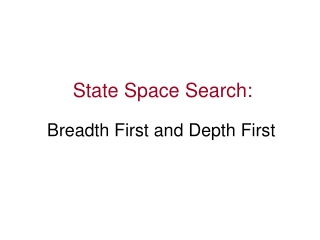DownloadDownload PresentationState Space Search:

# State Space Search:

Télécharger la présentation## State Space Search:

- - - - - - - - - - - - - - - - - - - - - - - - - - - E N D - - - - - - - - - - - - - - - - - - - - - - - - - - -
##### Presentation Transcript

1. State Space Search: Breadth First and Depth First

2. Motivations • Many problems can be viewed as reaching a goal state from a given starting point, e.g., the farmer-wolf-goat-cabbage problem. • Often there is an underlying state space successor function to proceed from one state to the next. • Search strategies are important methods to explore such a space to obtain the goal state.

3. Objectives • Top down search • Bottom up search • Breadth first search • Depth first search • Compare breadth first and depth first

4. State space for tic-tac-toe game • Represent a problem as a state space • Nodes represent discrete states, e.g., configuration of game board. • Arcs (links, edges) represent transition between states e.g., move in a game. • Analyze the structure and complexity of problem and search procedures using graph theory.

5. A B C D E F G H I J K L M N O P Q Breadth-first searching • A breadth-first search (BFS) explores nodes nearest the root before exploring nodes further away • For example, after searching A, then B, then C, the search proceeds with D,E,F,G • Node are explored in the order A B C D E F G H I J K L M N O P Q • J will be found before N

6. Breadth-first search algorithm Nodes are lining up to be visited in open.closed keeps track of all the nodes visited already.

7. A trace of breadth-first algorithm B is not the goal.Put his children onto the queue.Put him in closed. He is done. | | | Items between red bars are siblings. | | | | | | | | | | | | goal is reached or open is empty.

8. Progress at iteration 6 open contains the nodes whose children have not been looked at yet. The queue is also called the frontier of the search. closed contains the nodes that have been visited, i.e., nodes that have been opened or expanded. | | | | | | | | | | | | | | |

9. A B C D E F G H I J K L M N O P Q Breadth-first searching summary

10. A B C D E F G H I J K L M N O P Q Depth-first searching • A depth-first search (DFS) explores a path all the way to a leaf before backtracking and exploring another path • For example, after searching A, thenB, then D, the search backtracks and tries another path from B • Node are explored in the order A B D E H L M N I O P C F G J K Q • N will be found before J

11. The depth-first search algorithm This is the only difference between depth-first and breadth-first.

12. A trace of depth-first algorithm top of stack

13. Snap shot at iteration 6 frontier visited

14. Recursive depth-first search algorithm • DFS(node v) { visit(v) for each child w of v, DFS(w)} • Recursion uses a stack to store data for previous calls. The children nodes (w’s)are placed on the undeclared (implicit) stack. • Breadth first uses a queue instead of a stack, no recursion. • At any given time, the top of the stack contains only the node on a path from the root to a goal. • The stack only needs to be large enough to hold the deepest search path. • When a goal node is found, the path can be extracted from the stack as the recursion unwinds.

15. Search sequences of depth-first and breadth-first Breadth first: A, B, C, … Depth first: 1, 2, 3, …

16. Comparing the ordering of search sequences • Determine the order of nodes (states) to be examined • Breadth-first search • When a state is examined, all of its children are examined, one after another • Explore the search space in a level-by-level fashion • Depth-first search • When a state is examined, all of its children and their descendants are examined before any of its siblings • Go deeper into the search space where possible

17. Summary • Graph representation • Nodes are problem solution states • Arcs are steps in problem solving • State Space Search • Find a solution path from start state to goal state. • Determine complexity of problem • Many problems (TSP) have exponential complexity, impossible to search exhaustively • Strategies to search large space need heuristic to reduce space and time complexity